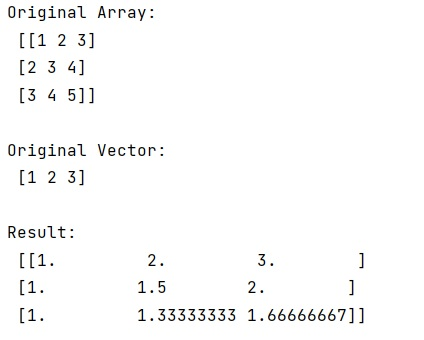# NumPy: Divide each row by a vector element

Given a NumPy array, we have to divide each row by a vector element.
Submitted by Pranit Sharma, on December 24, 2022

NumPy is an abbreviated form of Numerical Python. It is used for different types of scientific operations in python. Numpy is a vast library in python which is used for almost every kind of scientific or mathematical operation. It is itself an array which is a collection of various methods and functions for processing the arrays.

## Dividing each row by a vector element

Suppose we are given a multidimensional NumPy array and also, we have a corresponding vector, we need to perform an operation on the NumPy array in such a way that each row is divided by the corresponding vector which means that each element of each row is divided by each element of the vector.

For this purpose, we will simply divide the whole NumPy array with the whole vector. A great way of doing this even for higher dimensional arrays.

Let us understand with the help of an example,

## Python code to divide each row by a vector element

```# Import counter from collections
from collections import Counter

# Import numpy
import numpy as np

# Creating a numpy array
arr = np.array([[1,2,3],[2,3,4],[3,4,5]])

# Display original array
print("Original Array:\n",arr,"\n")

# Creating a vector
vec = np.array([1,2,3])

# Display original vector
print("Original Vector:\n",vec,"\n")

# Dividing arr by vec
res = arr/vec[:,None]

# Display result
print("Result:\n",res,"\n")
```

Output: